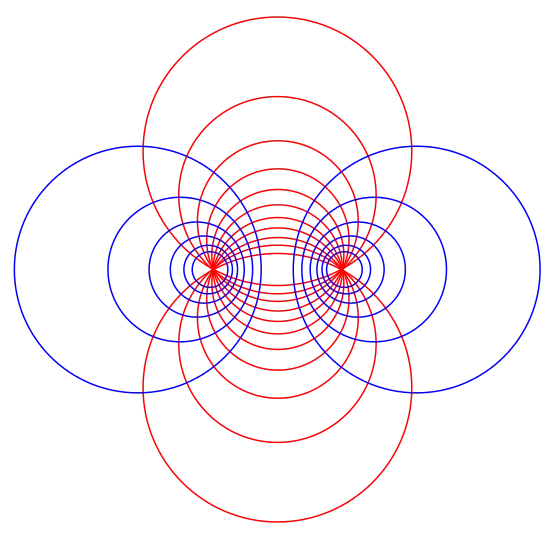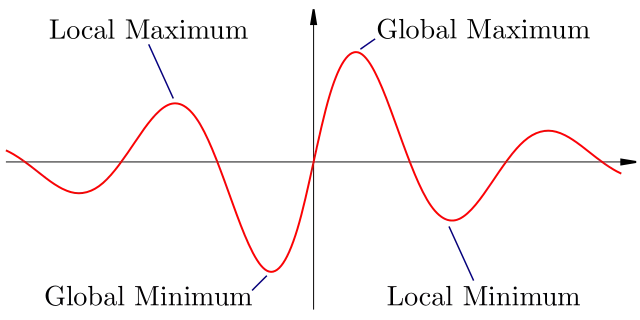# Category Mathematics

## Differential Equations Class 12 notes | IIT-JEE study materialSo, in calculus we are starting the topic of differential equations, we will be seeing what does a differential equation exactly means, what are partial differential equations, the significance of differential equations in various disciplines is very vast, we will…

## Applications of Derivatives class 12 revision notes | IIT-JEE notes, study materialWe have analyzed the concept of derivative/Differentiation of a function, this concept is widely used in various fields like math, physics, engineering sciences, etc, derivatives are used to analyze any function by calculating its first and second derivative, Hence we…